# Graphical simultaneous equations

We know

Simultaneous equations =  two equations with two unknowns

and

Graphical = Draw the lines on the graph.

The only additional item you need to know is:When they meet they find the solution.

(The point where the two lines intersect on a graph is the solution).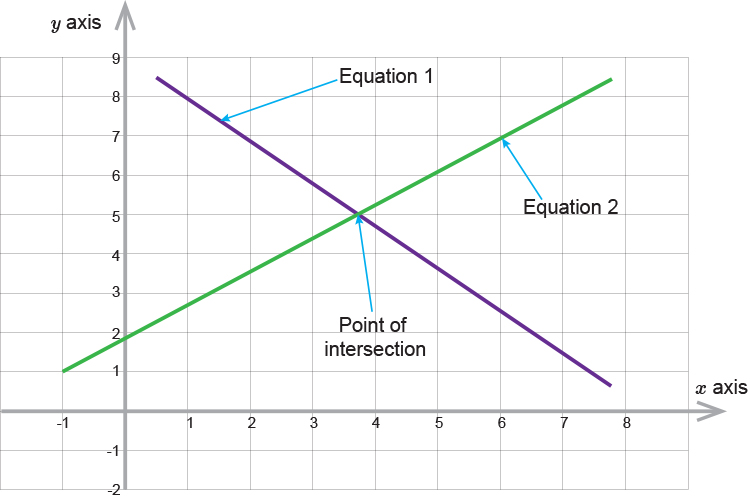If you find where x  and y  intersect on a graph that is the solution.

NOTE:

To continue you must understand our section on formula for a straight line.

That is:

1. Always make y  the subject of the formula
2. Formula of a straight line is y=mx+c
3. Plot x  and y  for given values of x

Example 1

Plot the graphs of the following equations and find their solution by finding the point of intersection.

2x+3y=6

4x-6y=-4

So first plot the graph of the following equation:

2x+3y=6

Subtract 2x  from both sides to get y  on its own.

2x-2x+3y=6-2x

3y=6-2x

Divide both sides by 3 to get y  on its own.

y=6/3-(2x)/3

y=2-2/3x

Lets plot some points on the graph

 If x=5 then y=2-2/3(5) =-1.33 x=4 then y=2-2/3(4) =-0.66 x=3 then y=2-2/3(3) =0 x=2 then y=2-2/3(2) =0.67 x=1 then y=2-2/3(1) =1.33 x=0 then y=2-2/3(0) =2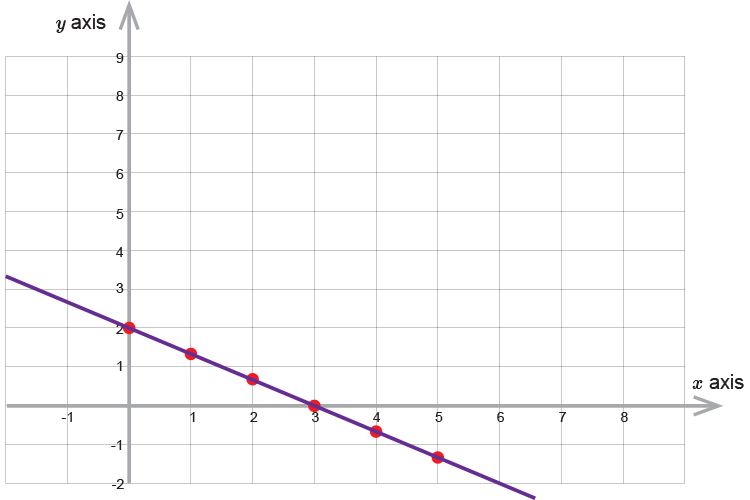Now lets add to the graph the equation:

4x-6y=-4

Subtract 4x  from both sides to get y  on its own.

-4x+4x-6y=-4-4x

-6y=-4-4x

Divide both sides by -6  to get y  on its own.

y=(-4)/(-6)-(4x)/-6

y=2/3+(2x)/3

Lets plot some points on the graph

 If x=5 then y=2/3+(2times5)/3 =2/3+10/3=12/3 =4 x=4 then y=2/3+(2times4)/3 =2/3+8/3=10/3 =3\1/3 x=3 then y=2/3+(2times3)/3 =2/3+6/3=8/3 =2\2/3 x=2 then y=2/3+(2times2)/3 =2/3+4/3=6/3 =2 x=1 then y=2/3+(2times1)/3 =2/3+2/3=4/3 =1\1/3 x=0 then y=2/3+(2times0)/3 =2/3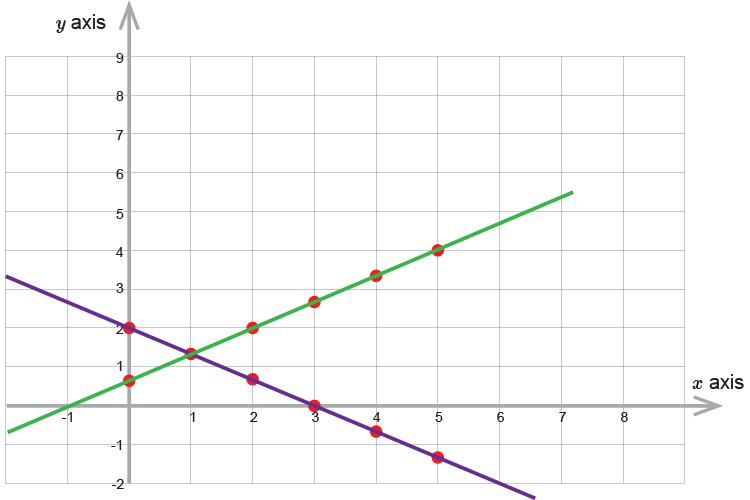We can see from the graph that the two lines intersect (and therefore the solution) at:

x=1           y=1\1/3

Example 2

Plot the graphs of the following equations and find their solution by finding the point of intersection.

y=x+3

x+y=7

So first plot the following equation on a graph.

y=x+3

Lets put some points on the graph

 If x=5 then y=5+3 =8 x=4 then y=4+3 =7 x=3 then y=3+3 =6 x=2 then y=2+3 =5 x=1 then y=1+3 =4 x=0 then y=0+3 =3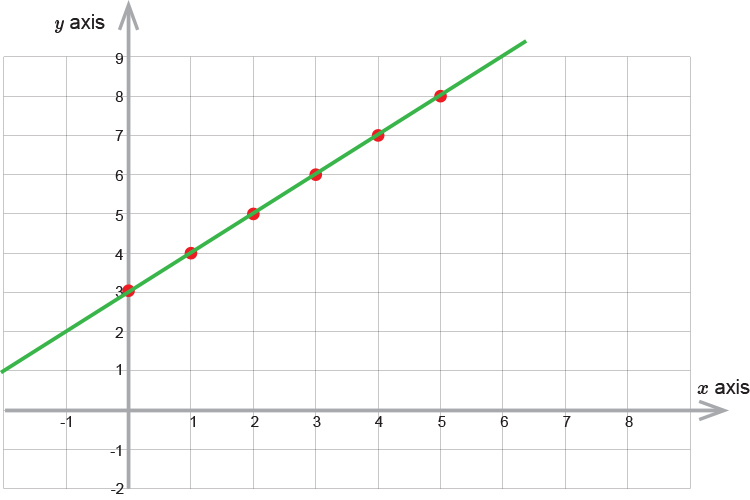Now lets add to the graph the equation:

x+y=7

Subtract x  from both sides to get y  on its own

x-x+y=7-x

y=7-x

Lets plot some points on the graph

 If x=5 then y=7-5 =2 x=4 then y=7-4 =3 x=3 then y=7-3 =4 x=2 then y=7-2 =5 x=1 then y=7-1 =6 x=0 then y=7-0 =7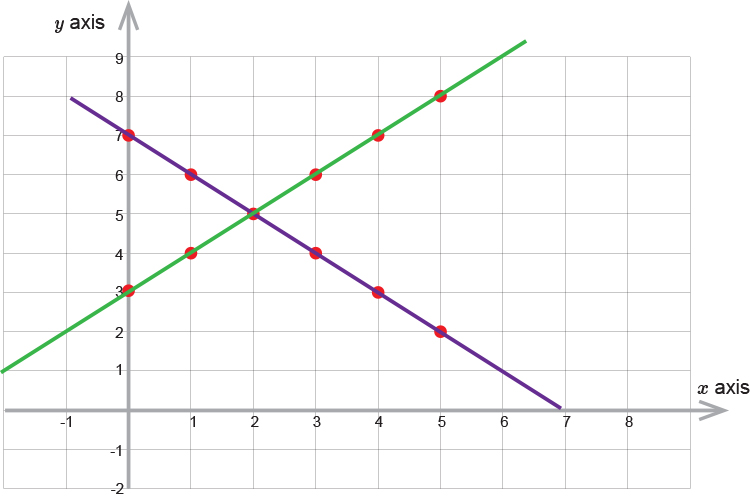We can see from the graph that the two line intersect (and therefore the solution) at:

x=2         y=5

Example 3

Plot the graphs of the following equations and find their solution by finding the point of intersection.

2x+2y=6

4x-6y=12

So first plot the graph of the following equation.

2x+2y=6

Subtract 2x  from both sides to get y  on its own

2x-2x+2y=6-2x

2y=6-2x

Divide both sides by 2 to get y  on its own

(2y)/2=6/2-(2x)/2

y=3-x

Lets put some points on the graph

 If x=5 then y=3-5 =-2 x=4 then y=3-4 =-1 x=3 then y=3-3 =0 x=2 then y=3-2 =1 x=1 then y=3-1 =2 x=0 then y=3-0 =3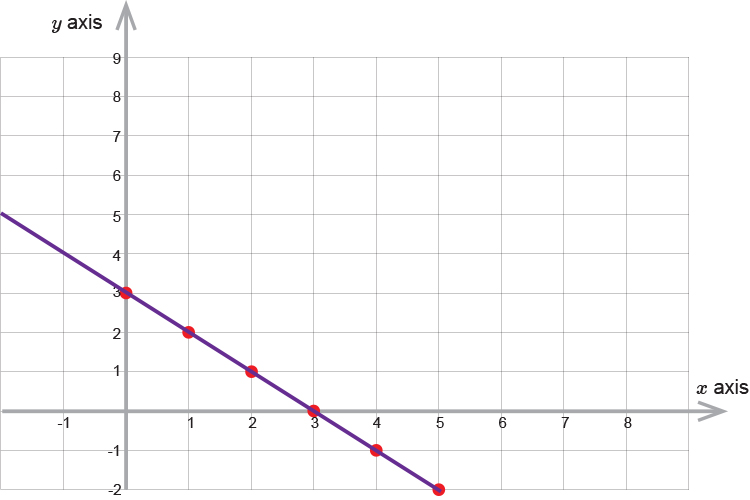Now lets add to the graph the equation:

4x-6y=12

Subtract 4x  from both sides to get y  on its own

4x-4x-6y=12-4x

-6y=12-4x

Divide both sides by -6  to get y  on its own.

(-6y)/(-6)=12/-6-(4x)/-6

y=-2+2/3x

Lets plot some points on the graph

 If x=5 then y=-2+2/3times5 =-2+10/3=-2+3\1/3 =1\1/3 x=4 then y=-2+2/3times4 =-2+8/3=-2+2\2/3 =2/3 x=3 then y=-2+2/3times3 =-2+6/3=-2+2 =0 x=2 then y=-2+2/3times2 =-2+4/3=-2+1\1/3 =-2/3 x=1 then y=-2+2/3times1 =-2+2/3=-2+2/3 =-1\1/3 x=0 then y=-2+2/3times0 =-2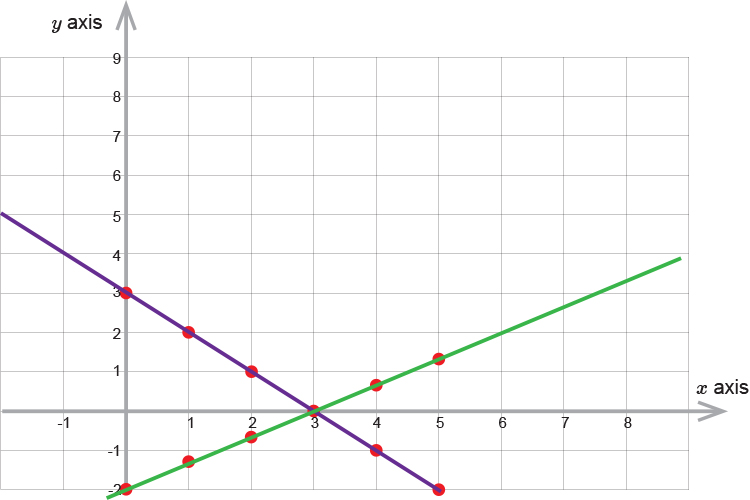We can see from the graph that the two line intersect (and therefore the solution) at:

x=3         y=0